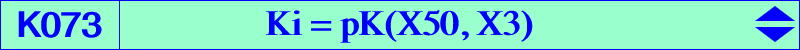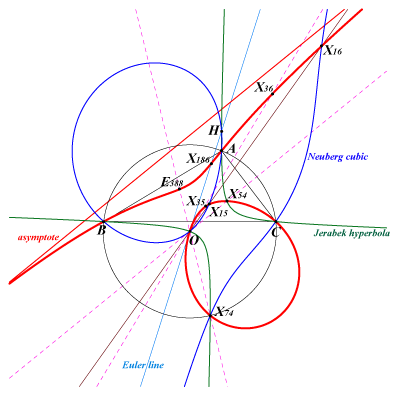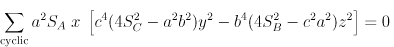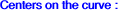X(3), X(15), X(16), X(35), X(36), X(54), X(74), X(186), X(1154), X(1511), X(3165), X(3166), X(3438), X(3439), X(6104), X(6105), X(14354), X(14367), X(14368), X(14369), X(37848), X(37850) i X(370), ig X(370) isogonal conjugates of the Ix-anticevian points. See Table 23.K073 is the inversive image of the Neuberg cubic in the circumcircle and the isogonal transform of the Kn cubic. See also Inverses of Isocubics. It is a circular pivotal cubic with pivot O = X(3) and pole X(50). The tangent at O is the Euler line. The singular focus is X(14652), the inverse of X(3448) in the circumcircle. It is anharmonically equivalent to the Neuberg cubic. See Table 20. K073 contains the midpoints of the segments joigning O and the three common points of the circumcircle and the Napoleon cubic. See a figure at Q011. Locus properties : K073 is the locus of point P such that the pedal triangles of P and its X(50)-isoconjugate P* are orthologic. It is the only circular cubic of this type. See CL021. Denote by A'B'C' the reflection triangle of P in the sidelines of ABC and by A", B", C" the reflections of A, B, C in the sidelines of A'B'C'. The triangles A'B'C' and A"B"C" are perspective (at Q) if and only if P lies on K073 (Paul Yiu, Hyacinthos #8631). The locus of Q is Q110. Locus of isopivots of circular pKs which pass through the isodynamic points X(15), X(16). The locus of the poles is K1049 = pK(X19627, X6) and the locus of the pivots is K001. See K735 for another locus property. Let ABC be a triangle and P a point in its plane. The perpendicular through A to AP intersects the circumcircle at A'. Let (Da) be the directrix of the parabola, (Pa), with focus A and tangent to the lines A'B, A'C. The lines (Db), (Dc) and the parabolas (Pb), (Pc) are defined cyclically. The lines (Da), (Db), (Dc) are concurrent (at Q) if and only if P lies on the cubic K073, and then Q lies on K001. Q is the inverse in (O) of the X(50)-isoconjugate of P, hence O, P, Q are collinear. (Pa), (Pb), (Pc) are also tangent at A1, B1, C1 to the sidelines BC, CA, AB respectively. These points A1, B1, C1 are collinear on (L) if and only if P lies on K004, and then the trilinear pole of (L) lies on K002 (Angel Montesdeoca, 2023-03-17).